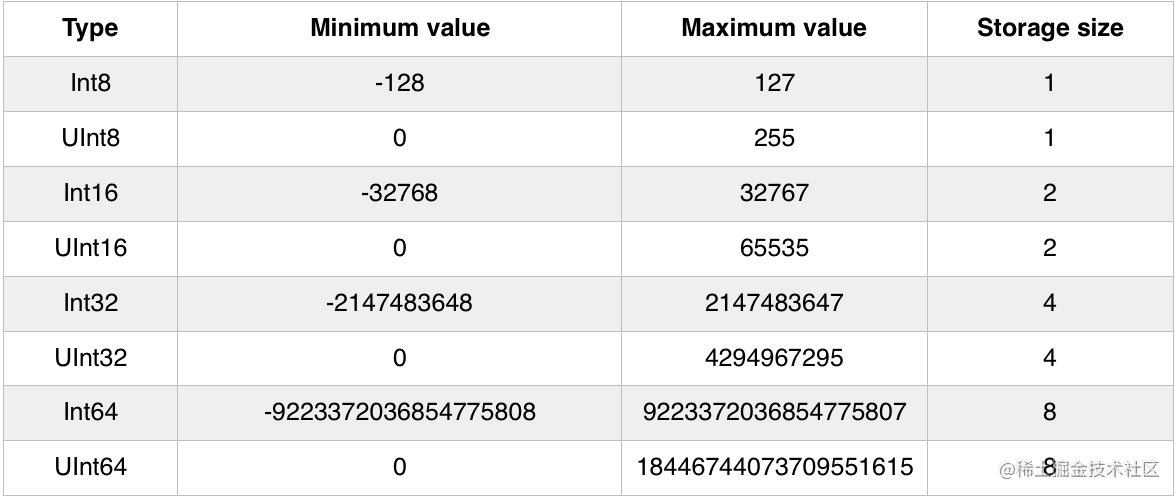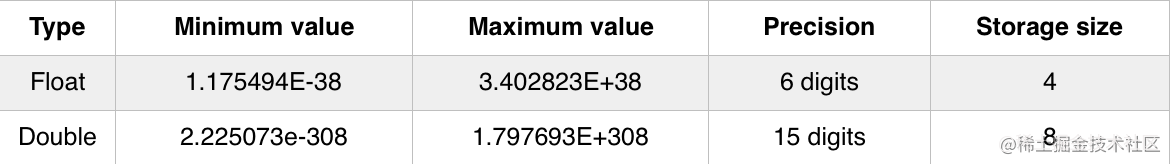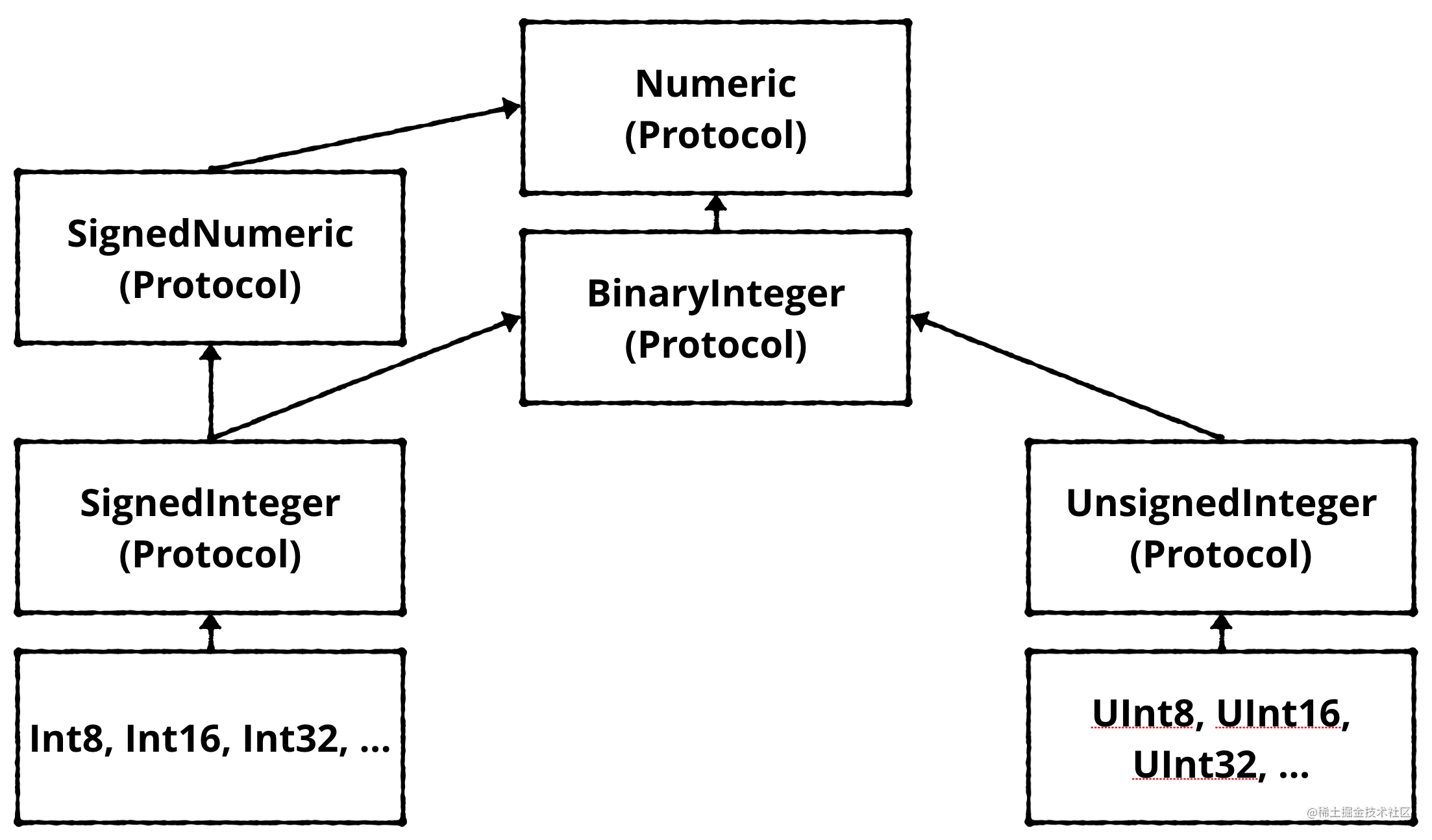# 二 类型与操作

### 1: 类型转换

``````var integer: Int = 100
var decimal: Double = 12.5
integer = decimal

``````Cannot assign value of type 'Double' to type 'Int'

``````integer = Int(decimal)

### 2: 混合类型的操作符

``````let hourlyRate: Double = 19.5
let hoursWorked: Int = 10
let totalCost: Double = hourlyRate * hoursWorked

``````Binary operator '*' cannot be applied to operands of type 'Double' and 'Int'

Swift要求您明确表示`Int`与Double相乘的含义，因为结果只能是一种类型。您想要结果为`Int`型，在执行乘法之前将Double型转换为`Int`型吗?或者你想要结果是一个Double型，在执行乘法之前将`Int`转换为`Double`型?

``````let totalCost: Double = hourlyRate * Double(hoursWorked)

##### 3: 类型推断

``````let typeInferredInt = 42

##### 4. Strings

####### 4.1 Characters and strings 字符数据类型可以存储单个字符。例如:

``````let characterA: Character = "a"

``````let characterDog: Character = "🐶"

``````let stringDog: String = "Dog"

``````let stringDog = "Dog" // Inferred to be of type String

####### 4.2 字符串拼接

``````var message = "Hello" + " my name is "
let name = "Matt"
message += name // "Hello my name is Matt

``````let exclamationMark: Character = "!"
message += String(exclamationMark) // "Hello my name is Matt!”

####### 4.3 字符串插入

``````message = "Hello my name is \(name)!" // "Hello my name is Matt!”

``````let oneThird = 1.0 / 3.0
let oneThirdLongString = "One third is \(oneThird) as a decimal.

``````One third is 0.3333333333333333 as a decimal.

###### 4.4 多行字符串

Swift有一种简洁的方式来表达包含多行字符串。当您需要在代码中放入一个非常长的字符串时，这可能非常有用。 你这样做:

``````let bigString = """
You can have a string
that contains multiple
lines
by
doing this.
"""
print(bigString)

``````You can have a string
that contains multiple
lines
by
doing this.

##### 5. 元组

``````let coordinates: (Int, Int) = (2, 3)

``````let coordinates = (2, 3)

``````let coordinatesDoubles = (2.1, 3.5)
// Inferred to be of type (Double, Double)

``````let coordinatesMixed = (2.1, 3)
// Inferred to be of type (Double, Int)

``````let x1 = coordinates.0
let y1 = coordinates.1

``````let coordinatesNamed = (x: 2, y: 3)
// Inferred to be of type (x: Int, y: Int)

``````let x2 = coordinatesNamed.x
let y2 = coordinatesNamed.y

``````let coordinates3D = (x: 2, y: 3, z: 1)
let (x3, y3, z3) = coordinates3D

``````let coordinates3D = (x: 2, y: 3, z: 1)
let x3 = coordinates3D.x
let y3 = coordinates3D.y
let z3 = coordinates3D.z

``````let (x4, y4, _) = coordinates3D

##### 五:一大堆数字类型``````let a: Int16 = 12
let b: UInt8 = 255
let c: Int32 = -100000

let answer = Int(a) + Int(b) + Int(c)  // answer is an Int

##### 六: Type aliases（类型别名）

Swift的一个有用功能就是能够创建自己的类型，这实际上是另一种类型的别名。这意味着你可以给你的类型一个更有用的名称来描述它是什么，但实际上它下面只是另一个类型。这称为类型别名。

``````typealias Animal = String

``````let myPet: Animal = "Dog"

``````typealias Coordinates = (Int, Int)
let xy: Coordinates = (2, 4)

##### 七 Protocols

Swift真正伟大的特性之一是，它通过所谓的协议将类型共性的概念形式化。通过学习该协议，您可以立即理解使用该协议的整个类型家族是如何工作的。Swift是第一种基于协议的语言。当您开始理解这些类型背后的协议时，您可以以其他语言无法实现的方式利用该系统。